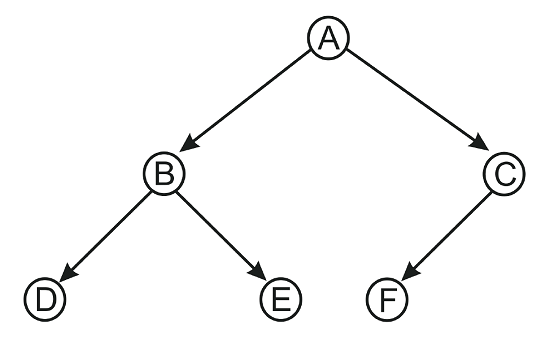# Binary Tree

## Binary Tree is fundamental data structure, where we can easily store and retrieve data. It is made up of nodes, where each node contains left pointer, right pointer and data.

Root pointer points to the top most node of the tree and the left and right pointers point to the smaller subtrees on either sides.

## Algorithm

1. First create a new node
Define a node having some data, references to its left and right child nodes.
2. Make the first value that you insert as root.
3. If you want to insert the next value to the left of root, insert as root->left, or if you want to insert to right insert as root->right
4. Repeat step 3 to insert the node in the right poisition in the Tree. For example, to insert the node to the left left of the root, insert as root->left->left.

### Example## C++ Program

```#include <stdlib.h>
#include <stdio.h>

struct node
{
char data;
struct node *left;
struct node *right;
};
struct node* newNode(char data)
{
// Allocate memory for new node
struct node* node = (struct node*)malloc(sizeof(struct node));

// Assign data to this node
node->data = data;

// Initialize left and right children as NULL
node->left = NULL;
node->right = NULL;
return(node);
}
void prinTree(struct node* node)	//Using PreOrder Traversal for Printing a Tree
{
if(node == NULL)
return;
printf("%c\n",node->data );
prinTree(node->left);
prinTree(node->right);

}

int main()
{				//Creating a Tree with nodes A, B, C, D, E, F
struct node *root = newNode('A'); // Creating a root with left and right pointers Null

root->left	 = newNode('B');    // Creating a left node to A
root->right	 = newNode('C');	// Creating a right node to A

root->left->left = newNode('D');    //Creating a left left node to A
root->left->right = newNode('E');	//Creating a left right node to A
root->right->left = newNode('F');	//Creating a right left node to A
prinTree(root);  //It will print the Tree
return 0;
}```

Translate »